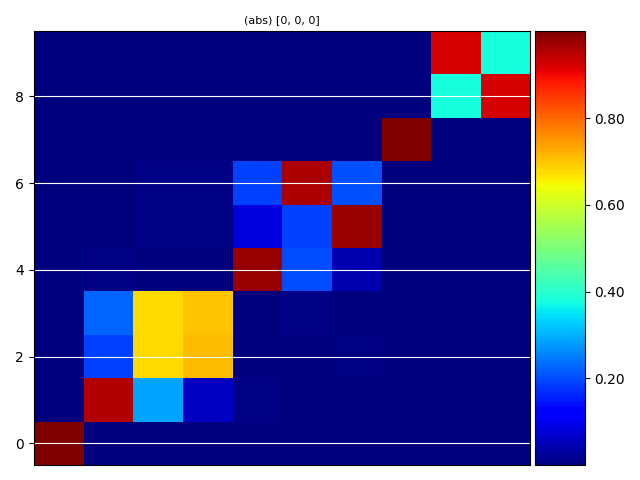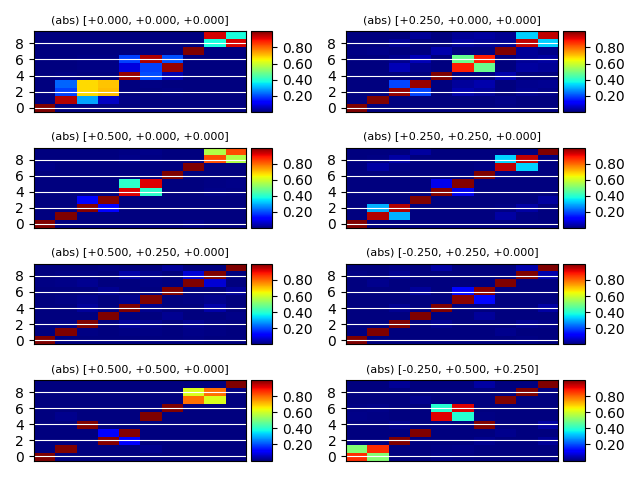# self-consistent GW¶

This example shows how to visualize the SCGW QP amplitudes in the KS basis set.

••Out:

/Users/gmatteo/git_repos/pymatgen/pymatgen/util/plotting.py:550: UserWarning: Matplotlib is currently using agg, which is a non-GUI backend, so cannot show the figure.
plt.show()
/Users/gmatteo/git_repos/pymatgen/pymatgen/util/plotting.py:550: UserWarning: Matplotlib is currently using agg, which is a non-GUI backend, so cannot show the figure.
plt.show()


import abipy.data as abidata
from abipy.abilab import abiopen

sigres = abiopen(abidata.ref_file("QPSC_SIGRES.nc"))

#print("calctyp",sigres.gwcalctyp)
#sigres.print_qps()

#qp = sigres.get_qpcorr(spin=0, kpoint=(0,0,0), band=0)
#print(qp)

# Visualize <KS_b|QP_b'> matrix at the Gamma point
sigres.plot_eigvec_qp(kpoint=[0, 0, 0])

# Plot <KS_b|QP_b'> components for all k-points in the SIGRES.
sigres.plot_eigvec_qp()


Total running time of the script: ( 0 minutes 1.159 seconds)

Gallery generated by Sphinx-Gallery